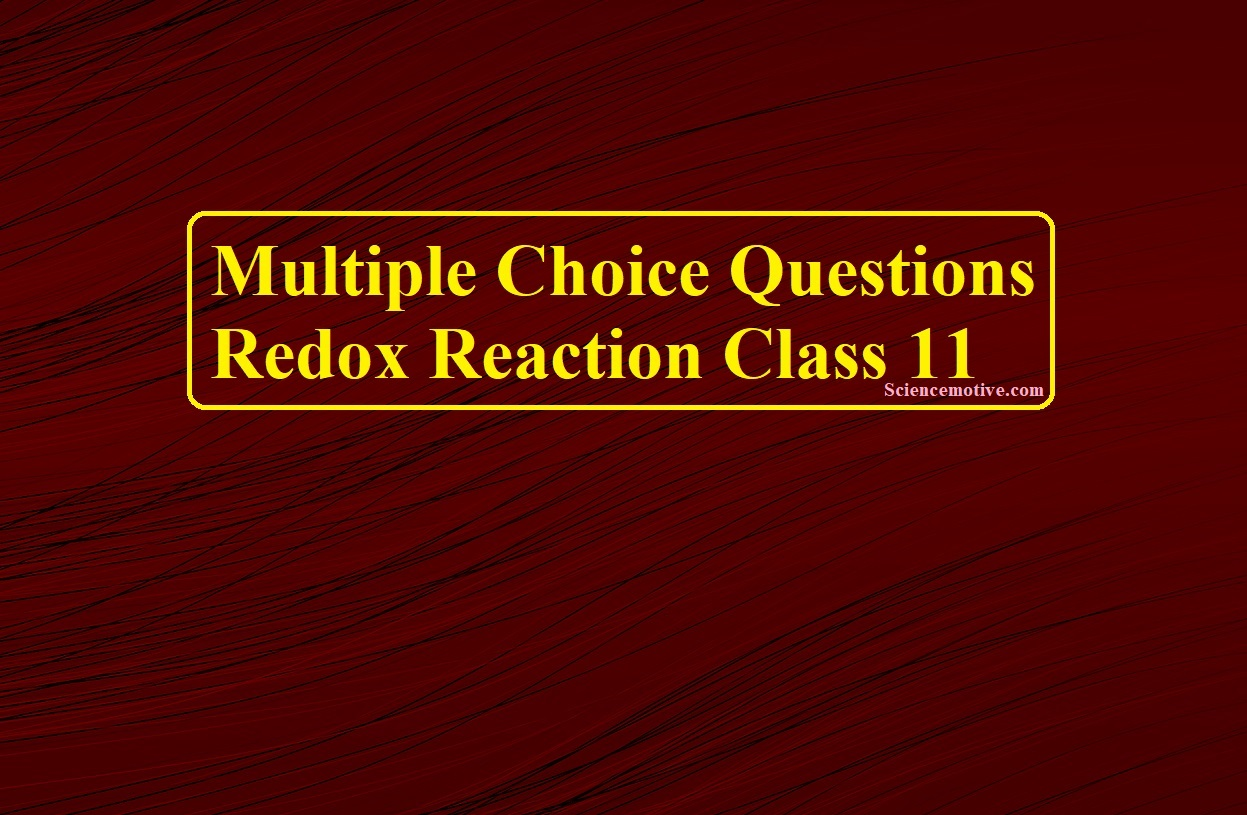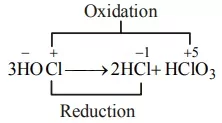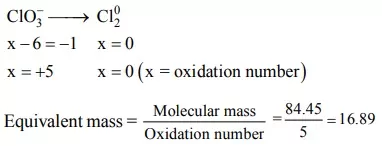# Multiple Choice Questions Redox Reaction Class 11# Multiple Choice Questions Redox Reaction Class 11

Multiple Choice Questions Redox Reaction Class 11

Que 1. Among NH3, HNO3, NaN3 and Mg3N2 the number of molecules having nitrogen in negative oxidation state is
(a) 1                             (b) 2                            (c) 3                             (d) 4
Ans 1.  (c) Calculating the oxidation state of nitrogen in given molecules
Oxidation state of N in NH3 is x + 3 × (+ 1) = 0 or x = – 3
Oxidation state on N in NaNO3 is 1 + x + 3 × (– 2) = 0 or x = + 5
Oxidation state of N in NaN3 is + 1 + 3x = 0 or x = – 1/3
Oxidation state of N in Mg3N2 is 3 × 2 + 2x = 0 or x = –3
Thus 3 molecules (i.e. NH3, NaN3 and Mg3N2) have nitrogen in negative oxidation state

­­­­­­­­Que 2. Fill up the table from the given choice.
Element                                  Oxidation number
Oxygen                                   –2 in most compounds (i) in H2O2 and (ii) in OF2
Halogen                                  –1 for (iii) in all its compounds
Hydrogen                                (iv) in most of its compounds (v) in binary metallic hydrides
Sulphur                                   (vi) in all sulphides
(i)              (ii)               (iii)              (iv)             (v)               (vi)
(a)      +1               +1                Cl               +1              –1                +2
(b)      –1              +2                F                +1              –1                 –2
(c)       –1             +1                F                +1              +2                +2
(d)       +1             +2               Cl               +1              +1                +6
Ans 2. (b)     –1    +2               F                +1              –1                –2

Que 3. The correct decreasing order of oxidation number of oxygen in compounds BaF2, O3, KO2 and OF2 is
(a) BaO2 > KO2 > O3 > OF2
(b) OF2 > O3 > KO2 > BaO2
(c) KO2 > OF2 > O3 > BaO2
(d) BaO2 > O3 > OF2 > KO2
Ans 3. (b) Oxidation no. of O are + 2, 0, – 1/2 and – 1 respectively

Que 4. Oxidation numbers of P in PO43–, of S in SO42– and that of Cr in Cr2O72– are respectively
(a) + 3, + 6 and + 5
(b) + 5, + 3 and + 6
(c) – 3, + 6 and + 6
(d) + 5, + 6 and + 6
Ans 4. (d)

Que 5. When Cl2 gas reacts with hot and concentrated sodium hydroxide solution, the oxidation number of chlorine changes from
(a) zero to +1 and zero to –5
(b) zero to –1 and zero to +5
(c) zero to –1 and zero to +3
(d) zero to +1 and zero to –3
Ans 5. (b) On reaction with hot and concentrated alkali a mixture of chloride and chlorate is formed.

Multiple Choice Questions Redox Reaction Class 11

Que 6.  Which of the following act as reducing agents?
(i) PO43–                      (ii) SO3                        (iii) PO32–                    (iv) NH3
(a) (i), (ii) and (iii)
(b) Only (iii)
(c) (i), (iii) and (iv)
(d) (iii) and (iv)
Ans 6. (d) In (i) and (ii) both P and S are in the highest oxidation state. In (iii) and (iv); P has an oxidation state of +4 which can be oxidized to a +5 state, while in the case of NH3 nitrogen has an oxidation state of –3 which can be oxidized.

Que 7. What products are expected from the disproportionation reaction of hypochlorous acid?
(a) HCl and Cl2O        (b) HCl and HClO3     (c) HClO3 and Cl2O    (d) HClO2 and HClO4
Ans 7. (b) During disproportionation same compound undergoes simultaneous oxidation-reduction.Que 8. In the disproportionation reaction 3HClO3 → HClO4 + Cl2 + 2O2 + H2O, the equivalent mass of the oxidizing agent is (molar mass of HClO3 = 84.45)
(a) 16.89                      (b) 32.22                     (c) 84.45                      (d) 28.15
Ans 8. (a)Que 9. Read the following passage and answer the questions that follow
Redox reactions are an important class of reactions that are taking place in our daily life. Metals are good reducing agents because they can lose electrons easily whereas non-metals are good oxidizing agents which can gain electrons easily. In electrolytic cells, electricity is passed to bring about a redox reaction. All rechargeable batteries act as electrolytic cells while recharging. Electrochemical cells produce electricity as a result of the redox reaction. A Salt bridge is used in an electrochemical cell to complete the internal circuit and prevent the accumulation of charges.
Que (i). What is an electrochemical cell?
Ans. The cell in which the chemical energy of redox reaction is converted into electrical energy.
Que (ii). Give one example of rechargeable cells widely used in vehicles.
Que (iii). Highly reactive metals are obtained by electrolysis of their molten ores, why?
Ans. It is because these metals are good reducing agents, cannot be obtained by chemical reduction.
Que (iv). What is the direction of the flow of current and electrons?
Ans. Electrons flow from anode to cathode whereas current flow from cathode to anode.
Que (v). What is standard electrode potential?
Ans. When the concentration of each species is unity, any gas involved is at 1 bar, the temperature is 298 K, the potential of the electrode is called standard electrode potential measured with respect to standard hydrogen electrode.

Multiple Choice Questions Redox Reaction Class 11

Que 10. Given E0
(i) Mg2+/Mg(s), E0 = – 2.36                           (ii) Ag+ /Ag(s), E0 = 0.80
(iii) Al3+/Al(s), E0 = – 1.66                             (iv) Cu2+/Cu(s), E0 = 0.52
Out of the above-given elements which is the strongest oxidizing agent and which is the weakest oxidizing agent?
(a) (iv) is strong whereas (ii) is the weakest oxidizing agent
(b) (ii) is the strongest whereas (i) is the weakest oxidizing agent
(c) (i) is the strongest whereas (ii) is the weakest oxidizing agent
(d) (ii) is the strongest whereas (iii) is the weakest oxidizing agent
Ans 10. (d) Negative E0 → Stronger reducing agent or weaker oxidizing agent
Positive E0 → Weaker reducing agent or stronger oxidizing agent

Que. 11. Arrange the following in the order of their decreasing electrode potentials: Mg, K, Ba and Ca
(a) K, Ca, Ba, Mg       (b) Ba, Ca, K, Mg       (c) Ca, Mg, K, Ba       (d) Mg, Ca, Ba, K
Ans 11. (d) The order of decreasing electrode potentials of Mg, K, Ba, and Ca is Mg > Ca > Ba > K

Que. 12. Which of the following sequences of T and F is correct for given statements. Here T stands for true and F stands for false statements
(i) Reducing agents lower the oxidation number of an element in a given substance. These reagents are also called reductants
(ii) Reducing agents are acceptors of electrons
(iii) Loss of electron(s) by any species is called oxidation reaction
(iv) Oxidation and reduction always occur simultaneously.
(a) TTTT                     (b) TFTT                     (c) TFFT                     (d) FTTT
Ans 12. (b) For statement (ii) reducing agents are donors of electrons.

Que 13. Which of the following elements does not show disproportionation tendency?
(a) Cl                           (b) Br                          (c) F                            (d) I
Ans 13. (c)

Multiple Choice Questions Redox Reaction Class 11